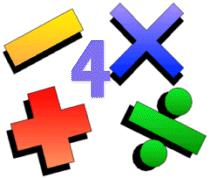Archives

Contribute

 For The Mathematically Inclined

Anil Saigal
07/22/2009

PROBLEM # 1
Using a balance scale and four weights you must be able to balance any integer load from 1 to 40. How much should each of the four weights weigh?

PROBLEM #2
A circle whose center is (1,r) is tangent to x-axis and to the curve y = x^2 in the first quadrant. Determine r to the nearest hundredth.

----------------------------------------------------------------------------------------------------------

Use Problem Solutions M-072309 as the subject line. Please include your full name in the text of the main message. Everyone with the right answer will be acknowledged in the next issue of Lokvani.

Please do not post your solution in Post Comments. No credit will be given for solutions not sent to anil@lokvani.com.

-------------------------------------------------------------------------------------------------------------

Problem Solutions 07/09/09

PROBLEM # 1

A bartender has a three pint glass and a five pint glass. A customer walks in and orders four pints of coke. Without a measuring cup but with an unlimited supply of coke how does he get four pints in either glass?

Solution:

Call the two glasses A (5 pints) and B (3). Fill B and pour into A twice leaving A and B with 5 and 1 pint resp. Now empty A. Pour out contents of B (1 pint) into A. Now fill out B and empty its contents (3 pints) into glass A. Now Glass A contains 4 pints as required.

PROBLEM #2

Let x be in radians with 0<x<pi/2. If sin(2sin(x)) = cos(2cos(x)), then tan(x) + cot(x) can be written as a / (b - pi^c) where a, b, and c are positive integers. Compute the sum (a+b+c).

Solution:

50.

Congratulations to Suman Luthra, Prabhakar Nadkarni andNikhil Nathwani, who were the winner of the last set of puzzles.

|